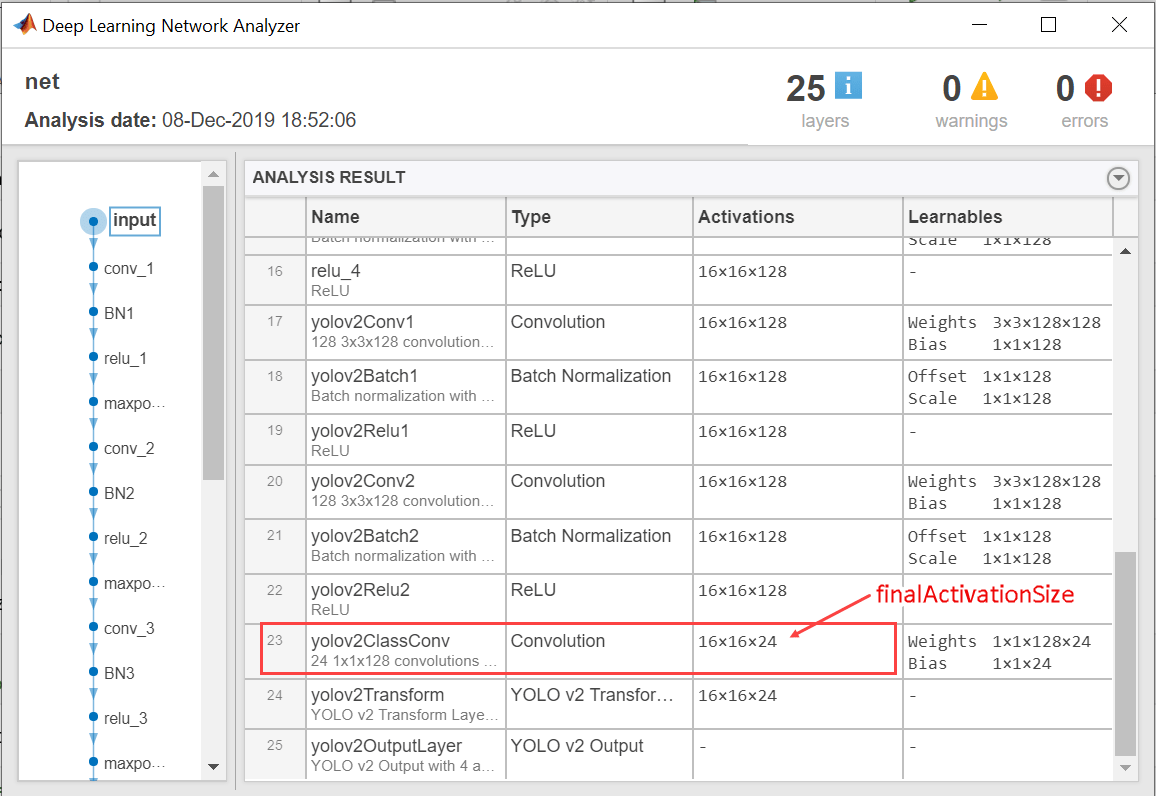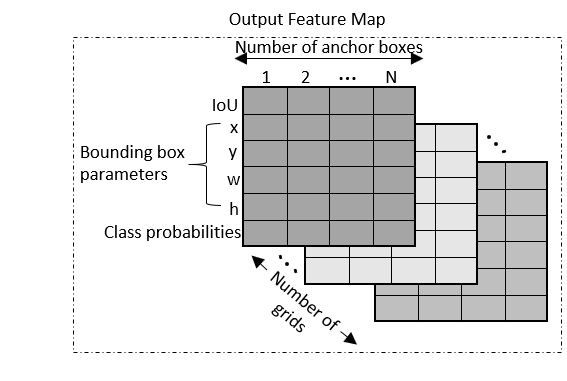Export YOLO v2 Object Detector to ONNX

This example shows how to export a YOLO v2 object detection network to ONNX™ (Open Neural Network Exchange) model format. After exporting the YOLO v2 network, you can import the network into other deep learning frameworks for inference. This example also presents the workflow that you can follow to perform inference using the imported ONNX model.

Export YOLO v2 Network

Export the detection network to ONNX and gather the metadata required to generate object detection results.

First, load a pretrained YOLO v2 object detector into the workspace.

net = input.detector.Network;

Next, obtain the YOLO v2 detector metadata to use for inference. The detector metadata includes the network input image size, anchor boxes, and activation size of last convolution layer.

Read the network input image size from the input YOLO v2 network.

inputImageSize = net.Layers(1,1).InputSize;

Read the anchor boxes used for training from the input detector.

anchorBoxes = input.detector.AnchorBoxes;

Get the activation size of the last convolution layer in the input network by using the analyzeNetwork function.

analyzeNetwork(net);finalActivationSize = [16 16 24];

Export to ONNX Model Format

Export the YOLO v2 object detection network as an ONNX format file by using the exportONNXNetwork function. Specify the file name as yolov2.onnx. The function saves the exported ONNX file to the current working folder.

filename = 'yolov2.onnx';
exportONNXNetwork(net,filename);

The exportONNXNetwork function maps the yolov2TransformLayer (Computer Vision Toolbox) and yolov2OutputLayer (Computer Vision Toolbox) in the input YOLO v2 network to the basic ONNX operator and identity operator, respectively. After you export the network, you can import the yolov2.onnx file into any deep learning framework that supports ONNX import.

Using the exportONNXNetwork, requires Deep Learning Toolbox™ and the Deep Learning Toolbox Converter for ONNX Model Format support package. If this support package is not installed, then the function provides a download link.

Object Detection Using Exported YOLO v2 Network

When exporting is complete, you can import the ONNX model into any deep learning framework and use the following workflow to perform object detection. Along with the ONNX network, this workflow also requires the YOLO v2 detector metadata inputImageSize, anchorBoxes, and finalActivationSize obtained from the MATLAB workspace. The following code is a MATLAB implementation of the workflow that you must translate into the equivalent code for the framework of your choice.

Preprocess Input Image

Preprocess the image to use for inference. The image must be an RGB image and must be resized to the network input image size, and its pixel values must lie in the interval [0 1].

resizedI = imresize(I,inputImageSize(1:2));
rescaledI = rescale(resizedI);

Pass Input and Run ONNX Model

Run the ONNX model in the deep learning framework of your choice with the preprocessed image as input to the imported ONNX model.

Extract Predictions from Output of ONNX Model

The model predicts the following:

• Intersection over union (IoU) with ground truth boxes

• x, y, w, and h bounding box parameters for each anchor box

• Class probabilities for each anchor box

The output of the ONNX model is a feature map that contains the predictions and is of size predictionsPerAnchor-by-numAnchors-by-numGrids.

• numAnchors is the number of anchor boxes.

• numGrids is the number of grids calculated as the product of the height and width of the last convolution layer.

• predictionsPerAnchor is the output predictions in the form [IoU;x;y;w;h;class probabilities].• The first row in the feature map contains IoU predictions for each anchor box.

• The second and third rows in the feature map contain predictions for the centroid coordinates (x,y) of each anchor box.

• The fourth and fifth rows in the feature map contain the predictions for the width and height of each anchor box.

• The sixth row in the feature map contains the predictions for class probabilities of each anchor box.

Compute Final Detections

To compute final detections for the preprocessed test image, you must:

• Rescale the bounding box parameters with respect to the size of the input layer of the network.

• Compute object confidence scores from the predictions.

• Obtain predictions with high object confidence scores.

• Perform nonmaximum suppression.

As an implementation guide, use the code for yolov2PostProcess function in Postprocessing Functions.

[bboxes,scores,labels] = yolov2PostProcess(featureMap,inputImageSize,finalActivationsSize,anchorBoxes);

Display Detection Results

Idisp = insertObjectAnnotation(resizedI,'rectangle',bboxes,scores);
figure
imshow(Idisp)

References

 Redmon, Joseph, and Ali Farhadi. “YOLO9000: Better, Faster, Stronger.” In 2017 IEEE Conference on Computer Vision and Pattern Recognition (CVPR), 6517–25. Honolulu, HI: IEEE, 2017. https://doi.org/10.1109/CVPR.2017.690.

Postprocessing Functions

function  [bboxes,scores,labels] = yolov2PostProcess(featureMap,inputImageSize,finalActivationsSize,anchorBoxes)

% Extract prediction values from the feature map.
iouPred = featureMap(1,:,:);
xyPred = featureMap(2:3,:,:);
whPred = featureMap(4:5,:,:);
probPred = featureMap(6,:,:);

% Rescale the bounding box parameters.
bBoxes = rescaleBbox(xyPred,whPred,anchorBoxes,finalActivationsSize,inputImageSize);

% Rearrange the feature map as a two-dimensional matrix for efficient processing.
predVal = [bBoxes;iouPred;probPred];
predVal = reshape(predVal,size(predVal,1),[]);

% Compute object confidence scores from the rearranged prediction values.
[confScore,idx] = computeObjectScore(predVal);

% Obtain predictions with high object confidence scores.
[bboxPred,scorePred,classPred] = selectMaximumPredictions(confScore,idx,predVal);

% To get the final detections, perform nonmaximum suppression with an overlap threshold of 0.5.
[bboxes,scores,labels] = selectStrongestBboxMulticlass(bboxPred', scorePred', classPred','RatioType','Union','OverlapThreshold',0.5);

end
function bBoxes = rescaleBbox(xyPred,whPred,anchorBoxes,finalActivationsSize,inputImageSize)

% To rescale the bounding box parameters, compute the scaling factor by using the network parameters inputImageSize and finalActivationSize.
scaleY = inputImageSize(1)/finalActivationsSize(1);
scaleX = inputImageSize(2)/finalActivationsSize(2);
scaleFactor = [scaleY scaleX];

bBoxes = zeros(size(xyPred,1)+size(whPred,1),size(anchors,1),size(xyPred,3),'like',xyPred);
for rowIdx=0:finalActivationsSize(1,1)-1
for colIdx=0:finalActivationsSize(1,2)-1
ind = rowIdx*finalActivationsSize(1,2)+colIdx+1;
for anchorIdx = 1 : size(anchorBoxes,1)

% Compute the center with respect to image.
cx = (xyPred(1,anchorIdx,ind)+colIdx)* scaleFactor(1,2);
cy = (xyPred(2,anchorIdx,ind)+rowIdx)* scaleFactor(1,1);

% Compute the width and height with respect to the image.
bw = whPred(1,anchorIdx,ind)* anchorBoxes(anchorIdx,1);
bh = whPred(2,anchorIdx,ind)* anchorBoxes(anchorIdx,2);

bBoxes(1,anchorIdx,ind) = (cx-bw/2);
bBoxes(2,anchorIdx,ind) = (cy-bh/2);
bBoxes(3,anchorIdx,ind) = bw;
bBoxes(4,anchorIdx,ind) = bh;
end
end
end
end
function [confScore,idx] = computeObjectScore(predVal)
iouPred = predVal(5,:);
probPred = predVal(6:end,:);
[imax,idx] = max(probPred,[],1);
confScore = iouPred.*imax;
end
function [bboxPred,scorePred,classPred] = selectMaximumPredictions(confScore,idx,predVal)
% Specify the threshold for confidence scores.
confScoreId = confScore >= 0.5;
% Obtain the confidence scores greater than or equal to 0.5.
scorePred = confScore(:,confScoreId);
% Obtain the class IDs for predictions with confidence scores greater than
% or equal to 0.5.
classPred = idx(:,confScoreId);
% Obtain the bounding box parameters for predictions with confidence scores
% greater than or equal to 0.5.
bboxesXYWH = predVal(1:4,:);
bboxPred = bboxesXYWH(:,confScoreId);
end